# Probabilistic Programming 3: Regression & Classification¶

#### Goal¶

• Understand how to estimate regression parameters using Bayesian inference.
• Understand how to estimate classification parameters using Bayesian inference.

#### Materials¶

In :
using Pkg
Pkg.activate("workspace")
Pkg.instantiate();
IJulia.clear_output();


## Problem: Economic growth¶

In 2008, the credit crisis sparked a recession in the US, which spread to other countries in the ensuing years. It took most countries a couple of years to recover. Now, the year is 2011. The Turkish government is asking you to estimate whether Turkey is out of the recession. You decide to look at the data of the national stock exchange to see if there's a positive trend.

In :
using CSV
using DataFrames
using ProgressMeter
using Plots
pyplot();


### Data¶

We are going to start with loading in a data set. We have daily measurements from Istanbul, from the 5th of January 2009 until 22nd of February 2011. The dataset comes from an online resource for machine learning data sets: the UCI ML Repository.

In :
# Read CSV file

Out:

536 rows × 2 columns

dateISE
StringFloat64
15-Jan-090.0357537
26-Jan-090.0254259
37-Jan-09-0.0288617
48-Jan-09-0.0622081
59-Jan-090.00985991
612-Jan-09-0.029191
713-Jan-090.0154453
814-Jan-09-0.0411676
915-Jan-090.000661905
1016-Jan-090.0220373
1119-Jan-09-0.0226925
1220-Jan-09-0.0137087
1321-Jan-090.000864697
1422-Jan-09-0.00381506
1523-Jan-090.00566126
1626-Jan-090.0468313
1727-Jan-09-0.00663498
1828-Jan-090.034567
1929-Jan-09-0.0205282
2030-Jan-09-0.0087767
212-Feb-09-0.0259191
223-Feb-090.0152795
234-Feb-090.0185778
245-Feb-09-0.0141329
256-Feb-090.036607
269-Feb-090.0113532
2710-Feb-09-0.040542
2811-Feb-09-0.0221056
2912-Feb-09-0.0148884
3013-Feb-090.00702675

We can plot the evolution of the stock market values over time.

In :
# Count number of samples
time_period = 436:536
num_samples = length(time_period)

# Extract columns
dates_num = 1:num_samples
dates_str = df[time_period,1]
stock_val = df[time_period,2]

# Set xticks
xtick_points = Int64.(round.(range(1, stop=num_samples, length=5)))

# Scatter exchange levels
scatter(dates_num,
stock_val,
color="black",
label="",
ylabel="Stock Market Levels",
xlabel="time (days)",
xticks=(xtick_points, [dates_str[i] for i in xtick_points]),
size=(800,300))

Out: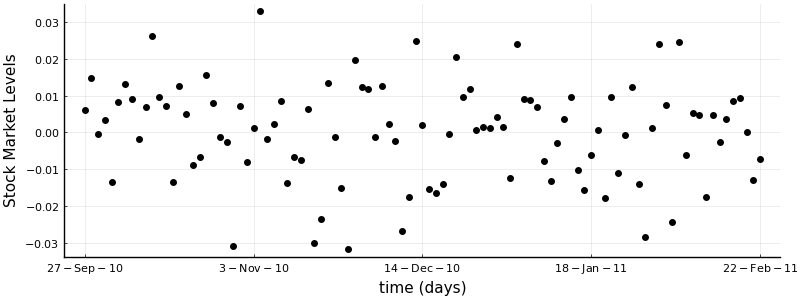### Model specification¶

We have dates $X$, referred to as "covariates", and stock exchange levels $Y$, referred to as "responses". A regression model has parameters $\theta$, used to predict $Y$ from $X$. We are looking for a posterior distribution for the parameters $\theta$:

$$\underbrace{p(\theta \mid Y, X)}_{\text{posterior}} \propto\ \underbrace{p(Y \mid X, \theta)}_{\text{likelihood}} \cdot \underbrace{p(\theta)}_{\text{prior}}$$

We assume each observation $Y_i$ is generated via:

$$Y_i = f_\theta(X_i) + e$$

where $e$ is white noise, $e \sim \mathcal{N}(0, \sigma^2_Y)$, and the regression function $f_\theta$ is linear: $f_\theta(X) = X \theta_1 + \theta_2$. The parameters consist of a slope coefficient $\theta_1$ and an intercept $\theta_2$, which are summarized into a parameter vector, $\theta = \begin{bmatrix}\theta_1 \\ \theta_2 \end{bmatrix}$. In practice, we augment the data matrix X with a 1, i.e., $\begin{bmatrix}X \\ 1 \end{bmatrix}$, so that we may define $f_\theta(X) = \theta^{\top}X$.

If we integrate out the noise $e$, then we obtain a Gaussian likelihood function centered on $f_\theta(X)$ with variance $\sigma^2_Y$:

$$Y_i \sim \mathcal{N}(f_\theta(X_i),\sigma^2_Y)\, \ .$$

Note that this corresponds to $p(Y \mid X, \theta)$. We know that the weights are real numbers and that they can be negative. That motivates us to use a Gaussian prior:

$$\theta \sim \mathcal{N}(\mu_\theta, \Sigma_\theta) \, .$$

Note that this corresponds to $p(\theta)$. For now, this is all we need. We're going to specify these two equations with ForneyLab. Our prior will become the first factor node: $f_a(\theta) = \mathcal{N}(\theta \mid \mu_\theta, \Sigma_\theta)$ and our likelihood becomes the second factor node $f_b(\theta) = \mathcal{N}(f_\theta(X_i),\sigma^2_Y)$. Note that $\theta$ is the only unknown variable, since $X$ and $Y$ are observed and $\mu_\theta$, $\Sigma_\theta$ and $\sigma^2_Y$ are clamped.

In :
using ForneyLab

In :
# Start factor graph
graph = FactorGraph();

# Prior weight parameters
μ_θ = [0., 0.]
Σ_θ = [1. 0.; 0. 1.]

# Noise variance
σ2_Y = 1.

# Add weight prior to graph
@RV θ ~ GaussianMeanVariance(μ_θ, Σ_θ, id=:f_a)

# Define covariates
@RV X

# Define likelihood
@RV Y ~ GaussianMeanVariance(dot(θ,X), σ2_Y, id=:f_b)

# Designate observed variables
placeholder(X, :X, dims=(2,))
placeholder(Y, :Y)

# Visualise the graph
ForneyLab.draw(graph)


But this is the graph for a single observation. In reality, we have multiple observations. If we want to consume all this information at once, the likelihood part of the above graph will need to be repeated.

In :
# Start factor graph
graph2 = FactorGraph();

# Prior weight parameters
μ_θ = [0., 0.]
Σ_θ = [1. 0.; 0. 1.]

# Noise variance
σ2_Y = 1.

# Add weight prior to graph
@RV θ ~ GaussianMeanVariance(μ_θ, Σ_θ, id=:f_a)

# Pre-define vectors for storing latent and observed variables
X = Vector{Variable}(undef, num_samples)
Y = Vector{Variable}(undef, num_samples)

for i = 1:num_samples

# Define i-th covariate
@RV X[i]

# Define likelihood of i-th response
@RV Y[i] ~ GaussianMeanVariance(dot(θ,X[i]), σ2_Y, id=Symbol("f_b"*string(i)))

# Designate observed variables
placeholder(X[i], :X, index=i, dims=(2,))
placeholder(Y[i], :Y, index=i);

end

# Visualise the graph
ForneyLab.draw(graph2)


It's hard to tell, but each of these likelihood nodes $f_{bi}$ is connected to the prior node $f_a$ via an equality node.

Now that we have our model, it is time to infer parameters.

In :
# Define and compile the algorithm
algorithm = messagePassingAlgorithm(θ)
source_code = algorithmSourceCode(algorithm)

# Evaluate the generated code to get the step! function
eval(Meta.parse(source_code));
# println(source_code)


Now, we iterate over time, feeding our data as it comes in and updating our posterior distribution for the parameters.

In :
# Initialize posterior
posterior = Dict()

data = Dict(:X => [[dates_num[i], 1] for i = 1:num_samples],
:Y => stock_val)

# Update posterior for θ
step!(data, posterior);


Let's visualize the resulting posterior.

In :
import ForneyLab: logPdf

# Define ranges for plot
x1 = range(-1.5, length=500, stop=1.5)
x2 = range(-2.5, length=500, stop=2.5)

# Draw contour plots of distributions
prior = ProbabilityDistribution(Multivariate, GaussianMeanVariance, m=μ_θ, v=Σ_θ)
p1a = contour(x1, x2, (x1,x2) -> exp(logPdf(prior, [x1,x2])), color="red", xlabel="θ1", ylabel="θ2", title="prior")
p1b = contour(x1, x2, (x1,x2) -> exp(logPdf(posterior[:θ], [x1,x2])), color="blue", xlabel="θ1", title="posterior")
plot(p1a, p1b, size=(800,300))

Out: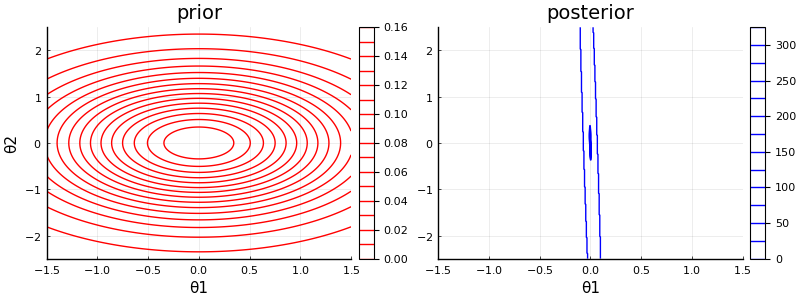It has become quite sharply peaked in a small area of parameter space.

We can use the MAP point estimate to compute and visualize the regression function $f_\theta$. The full predictive distribution is left for the PP Assignment.

In :
# Extract estimated weights
θ_MAP = mode(posterior[:θ])

# Report results
println("Slope coefficient = "*string(θ_MAP))
println("Intercept coefficient = "*string(θ_MAP))

# Make predictions
regression_estimated = dates_num * θ_MAP .+ θ_MAP;

Slope coefficient = -4.3204689272882614e-5
Intercept coefficient = 0.0022453122200430573


Let's visualize it.

In :
# Visualize observations
scatter(dates_num, stock_val, color="black", xticks=(xtick_points, [dates_str[i] for i in xtick_points]), label="observations", legend=:topleft)

# Overlay regression function
plot!(dates_num, regression_estimated, color="blue", label="regression", linewidth=2)

Out: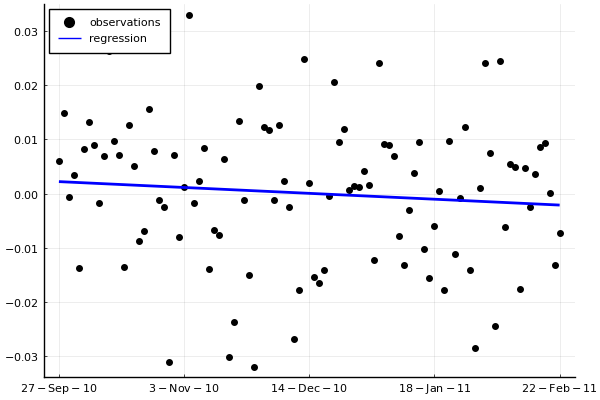The slope coefficient $\theta_1$ is negative and the plot shows a decreasing line. The ISE experienced a negative linear trend from October 2010 up to March 2011. Assuming the stock market is an indicator of economic growth, then we may conclude that in March 2011 the Turkish economy is still in recession.

#### $\ast$ Try for yourself¶

Change the time period variable. Re-run the regression and see how the results change.

### Recursive estimation¶

Our graph is quite large, which means our inference algorithm is slow. But as I already said earlier, the graph is essentially a repetition of the same structure. In this case, we don't need to generate such a large graph; we can recursively estimate the classification parameters. To do this, we essentially estimate parameters for a single observation, and make the resulting posterior our prior for the next observation.

Let's first re-define the subgraph for a single observation.

In :
# Start factor graph
graph = FactorGraph();

# Noise variance
σ2_Y = 1.

# Add weight prior to graph
@RV θ ~ GaussianMeanVariance(placeholder(:μ_θ, dims=(2,)),
placeholder(:Σ_θ, dims=(2,2)), id=:f_a)

# Define covariates
@RV X

# Define likelihood
@RV Y ~ GaussianMeanVariance(dot(θ,X), σ2_Y, id=:f_b)

# Designate observed variables
placeholder(X, :X, dims=(2,))
placeholder(Y, :Y)

# Visualise the graph
ForneyLab.draw(graph)

In :
# Define and compile the algorithm
algorithm = messagePassingAlgorithm(θ)
source_code = algorithmSourceCode(algorithm)

# Evaluate the generated code to get the step! function
eval(Meta.parse(source_code));


The syntax for compiling the inference algorithm is the same as before, but now we execute it differently. We feed in each sample and perform a step update for the posterior.

In :
# Initialize posteriors dictionary
posterior = Dict()
posterior[:θ] = ProbabilityDistribution(Multivariate, GaussianMeanVariance, m=[0.,0.], v=[1. 0.;0. 1.])

@showprogress for i = 1:num_samples

data = Dict(:X => [dates_num[i], 1],
:Y => stock_val[i],
:μ_θ => mean(posterior[:θ]),
:Σ_θ => cov(posterior[:θ]))

# Update posterior for θ
step!(data, posterior)
end

Progress: 100%|█████████████████████████████████████████| Time: 0:00:00


This is much faster. If we now visualize the resulting posterior, we see that it is not exactly the same. Recursive estimation is not mathematically equivalent to non-recursive estimation (it's the difference between filtering and smoothing, for those familiar). In this case, it produces a less sharply peaked posterior (note the y-axis between this plot and the previous posterior plot).

In :
import ForneyLab: logPdf

# Define ranges for plot
x1 = range(-2, length=500, stop=2)
x2 = range(-3, length=500, stop=3)

# Draw contour plots of distributions
prior = ProbabilityDistribution(Multivariate, GaussianMeanVariance, m=μ_θ, v=Σ_θ)
p1a = contour(x1, x2, (x1,x2) -> exp(logPdf(prior, [x1,x2])), color="red", xlabel="θ1", ylabel="θ2", title="prior")
p1b = contour(x1, x2, (x1,x2) -> exp(logPdf(posterior[:θ], [x1,x2])), color="blue", xlabel="θ1", title="posterior")
plot(p1a, p1b, size=(800,300))

Out: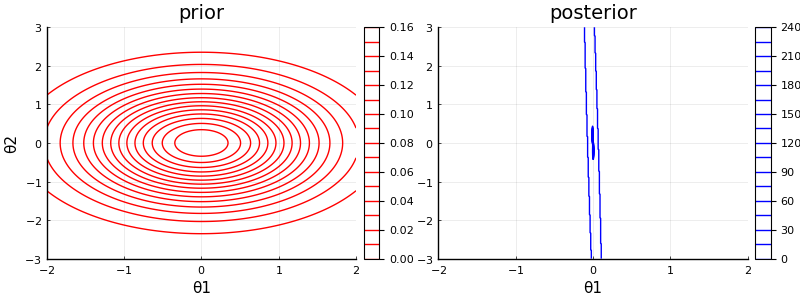## Problem: Credit Assignment¶

We will now look at a classification problem. Suppose you are a bank and that you have to decide whether you will grant credit, e.g. a mortgage or a small business loan, to a customer. You have a historic data set where your experts have assigned credit to hundreds of people. You have asked them to report on what aspects of the problem are important. You hope to automate this decision process by training a classifier on the data set.

### Data¶

The data set we are going to use actually comes from the UCI ML Repository. It consists of past credit assignments, labeled as successful (=1) or unsuccessful (=0). Many of the features have been anonymized for privacy concerns.

In :
# Read CSV file

# Split dataframe into features and labels
features_train = convert(Array, df[:,1:7])
labels_train = convert(Array, df[:,8]);

# Store number of features
num_features = size(features_train,2)

# Number of training samples
num_train = size(features_train,1);


Let's visualize the data and see if we can make sense of it.

In :
scatter(features_train[labels_train .== 0, 1], features_train[labels_train .== 0, 2], color="blue", label="unsuccessful", xlabel="feature1", ylabel="feature2")
scatter!(features_train[labels_train .== 1, 1], features_train[labels_train .== 1, 2], color="red", label="successful")

Out: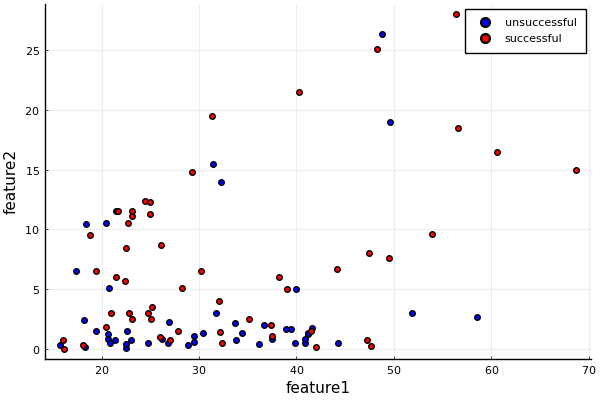Mmhh, it doesn't look like the samples can easily be separated. This will be challenging.

#### $\ast$ Try for yourself¶

The plot above shows features 1 and 2. Have a look at the other combinations of features.

### Model specification¶

We have features $X$, labels $Y$ and parameters $\theta$. Same as with regression, we are looking for a posterior distribution of the classification parameters:

$$\underbrace{p(\theta \mid Y, X)}_{\text{posterior}} \propto\ \underbrace{p(Y \mid X, \theta)}_{\text{likelihood}} \cdot \underbrace{p(\theta)}_{\text{prior}}$$

The likelihood in this case will be of a Logit form:

$$p(Y \mid X, \theta) = \prod_{i=1}^{N} \ \text{Logit}(Y_i \mid f_\theta(X_i), \xi_i) \, .$$

A "Logit" is short for a Bernoulli distribution with a sigmoid transfer function: $\sigma(x) = 1 / (1 + \exp(-x))$. The sigmoid maps the input to the interval $(0,1)$ so that the result acts as a rate parameter to the Bernoulli. Check Bert's lecture on discriminative classification for more information.

We are not using a Laplace approximation to the posterior, but rather a "local variational method" (see Section 10.5 of Bishop). We won't go into how that works here. All you need to know in this implementation is that there is a second parameter to the Logit, $\xi$ (the "local variational parameter"), which has to be estimated just as the classification parameters $\theta$ (but doesn't need a prior).

We will use a Gaussian prior distribution for the classification parameters $\theta$:

$$p(\theta) = \mathcal{N}(\theta \mid \mu_\theta, \Sigma_\theta) \, .$$

Note that the true posterior is still approximated with a Gaussian distribution.

In :
import LinearAlgebra: I

In :
# Start factor graph
graph = FactorGraph();

# Parameters for priors
μ_θ = zeros(num_features+1,)
Σ_θ = Matrix{Float64}(I, num_features+1, num_features+1)

# Define a prior over the weights
@RV θ ~ GaussianMeanVariance(μ_θ, Σ_θ)

X = Vector{Variable}(undef, num_train)
ξ = Vector{Variable}(undef, num_train)
Y = Vector{Variable}(undef, num_train)

for i = 1:num_train

# Features
@RV X[i]

# Local variational parameter
@RV ξ[i]

# Logit likelihood
@RV Y[i] ~ Logit(dot(θ, X[i]), ξ[i])

# Observed
placeholder(X[i], :X, index=i, dims=(num_features+1,))
placeholder(Y[i], :Y, index=i)

end


We will now compile an inference algorithm for this model. Since we now have two unknown variables, $\theta$ and $\xi$, we need to define a PosteriorFactorization(). With this function, we are basically telling ForneyLab that these variables need to be estimated separately (i.e., generate two step! functions).

In :
# We specify a recognition distribution
q = PosteriorFactorization(θ, ξ, ids=[:θ, :ξ])

# Define and compile the algorithm
algorithm = messagePassingAlgorithm()
source_code = algorithmSourceCode(algorithm)

# Bring the generated source code into scope
eval(Meta.parse(source_code));


Now that we have compiled the algorithm, we are going to iteratively update the classification parameters and the local variational parameter.

In :
# Initialize posteriors
posteriors = Dict()
for i = 1:num_train
posteriors[:ξ_*i] = ProbabilityDistribution(Function, mode=1.0)
end

data = Dict(:X => [[features_train[i,:]; 1] for i in 1:num_train],
:Y => labels_train)

@showprogress for i = 1:10

# Update classification parameters
stepθ!(data, posteriors)

# Update local variational parameters
stepξ!(data, posteriors)
end

Progress: 100%|█████████████████████████████████████████| Time: 0:00:14


### Predict test data¶

The bank has some test data for you as well.

In :
# Read CSV file

# Split dataframe into features and labels
features_test = convert(Array, df[:,1:7])
labels_test = convert(Array, df[:,8])

# Number of test samples
num_test = size(features_test,1);


You can classify test samples by taking the MAP for the classification parameters, computing the linear function $f_\theta$ and rounding the result to obtain the most probable label.

In :
import ForneyLab: unsafeMode

In :
# Extract MAP estimate of classification parameters
θ_MAP = unsafeMode(posteriors[:θ])

# Compute dot product between parameters and test data
fθ_pred = [features_test ones(num_test,)] * θ_MAP

# Predict labels
labels_pred = round.(1 ./(1 .+ exp.( -fθ_pred)));

# Compute classification accuracy of test data
accuracy_test = mean(labels_test .== labels_pred)

# Report result
println("Test Accuracy = "*string(accuracy_test*100)*"%")

Test Accuracy = 63.0%


Mmmhh... If you were a bank, you might decide that you don't want to automatically assign credit to your customers.